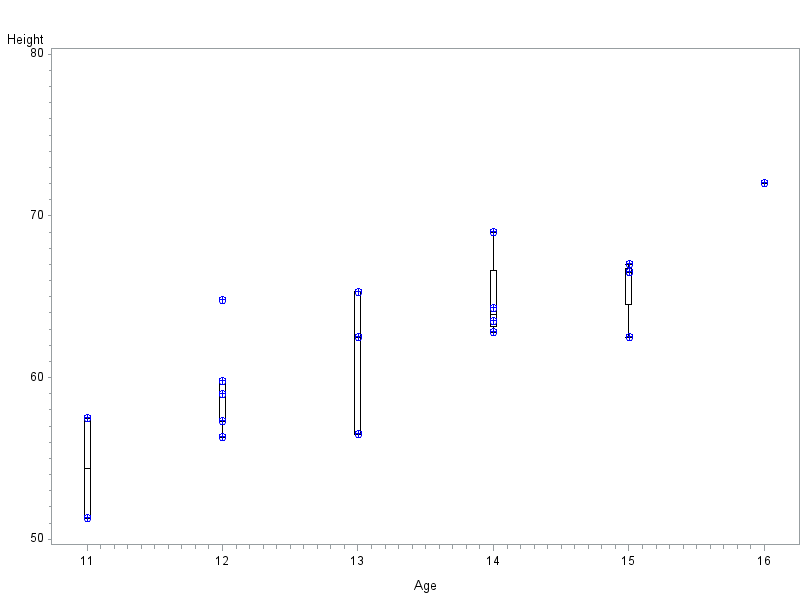## boxplot

i have used the guied for the first time to do a box plot and i cant find the opton to add the "+" for the mean , can anyone please help me with this ?

i see from the code that its not using proc boxplot and that its using gplot , maybe its not possible to add the "+" via gplot ?

is it normal that its using gplot ?

thanks

CL

## Re: boxplot

With gplot, you can do a boxplot plot, and then do a scatter plot (with the '+' character, or any character you want), and then 'overlay' them.  Here is the basic syntax (you can add more options to "fancy it up" a bit!)...

symbol1 interpol=boxcti color=black;
symbol2 interpol=none value=+ color=blue;

axis1 offset=(5,5);

proc gplot data=sashelp.class;
plot height*age=1 height*age=2 / overlay haxis=axis1;
run;Discussion stats